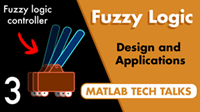# Get Started with Fuzzy Logic Toolbox

Design and simulate fuzzy logic systems

Fuzzy Logic Toolbox™ provides MATLAB® functions, apps, and a Simulink® block for analyzing, designing, and simulating fuzzy logic systems. The product lets you specify and configure inputs, outputs, membership functions, and rules of type-1 and type-2 fuzzy inference systems.

The toolbox lets you automatically tune membership functions and rules of a fuzzy inference system from data. You can evaluate the designed fuzzy logic systems in MATLAB and Simulink. Additionally, you can use the fuzzy inference system as a support system to explain artificial intelligence (AI)-based black-box models. You can generate standalone executables or C/C++ code and IEC 61131-3 Structured Text to evaluate and implement fuzzy logic systems.

## Tutorials

• What Is Fuzzy Logic?

Fuzzy logic uses linguistic variables, defined as fuzzy sets, to approximate human reasoning.

• Foundations of Fuzzy Logic

A fuzzy logic system is a collection of fuzzy if-then rules that perform logical operations on fuzzy sets.

• Fuzzy vs. Nonfuzzy Logic

To illustrate the value of fuzzy logic, examine both linear and fuzzy approaches to a basic tipping problem.

• Fuzzy Inference Process

Fuzzy inference maps an input space to an output space using a series of fuzzy if-then rules.

• Defuzzification Methods

Compare the defuzzification methods supported by Fuzzy Logic Toolbox software.

## VideosWhat Is Fuzzy Logic?
Fuzzy logic allows you to design a fuzzy inference system, which is a function that maps a set of inputs to outputs using human interpretable rules rather than more abstract mathematics.Fuzzy Inference System Walkthrough
A fuzzy inference system uses if-then rules, membership functions, and fuzzy operators to map a set of inputs to outputs.Fuzzy Logic Examples
Using experience and intuition, with no mathematical model, you can design a fuzzy logic controller that can balance a pole on a cart.